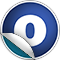# GeometryIncludes

### Related Docs: trait GeometryIncludes | package geometry

#### object GeometryIncludes extends GeometryIncludes

Source
GeometryIncludes.scala
Linear Supertypes
Ordering
1. Alphabetic
2. By Inheritance
Inherited
1. GeometryIncludes
2. GeometryIncludes
3. AnyRef
4. Any
1. Hide All
2. Show All
Visibility
1. Public
2. All

### Value Members

1. #### final def !=(arg0: Any): Boolean

Definition Classes
AnyRef → Any
2. #### final def ##(): Int

Definition Classes
AnyRef → Any
3. #### final def ==(arg0: Any): Boolean

Definition Classes
AnyRef → Any
4. #### final def asInstanceOf[T0]: T0

Definition Classes
Any
5. #### def clone(): AnyRef

Attributes
protected[java.lang]
Definition Classes
AnyRef
Annotations
@throws( ... )
6. #### final def eq(arg0: AnyRef): Boolean

Definition Classes
AnyRef
7. #### def equals(arg0: Any): Boolean

Definition Classes
AnyRef → Any
8. #### def finalize(): Unit

Attributes
protected[java.lang]
Definition Classes
AnyRef
Annotations
@throws( classOf[java.lang.Throwable] )
9. #### final def getClass(): Class[_]

Definition Classes
AnyRef → Any
10. #### def hashCode(): Int

Definition Classes
AnyRef → Any
11. #### final def isInstanceOf[T0]: Boolean

Definition Classes
Any
12. #### implicit def jfxBoundingBox2sfx(b: javafx.geometry.BoundingBox): BoundingBox

Converts a `javafx.geometry.BoundingBox` instance to its ScalaFX counterpart.

Converts a `javafx.geometry.BoundingBox` instance to its ScalaFX counterpart.

b

JavaFX BoundingBox

returns

ScalaFX BoundingBox

Definition Classes
GeometryIncludes
13. #### implicit def jfxBounds2sfx(b: javafx.geometry.Bounds): Bounds

Converts a `javafx.geometry.Bounds` instance to its ScalaFX counterpart.

Converts a `javafx.geometry.Bounds` instance to its ScalaFX counterpart.

b

JavaFX Bounds

returns

ScalaFX Bounds

Definition Classes
GeometryIncludes
14. #### implicit def jfxDimension2D2sfx(d: javafx.geometry.Dimension2D): Dimension2D

Converts a `javafx.geometry.Dimension2D` instance to its ScalaFX counterpart.

Converts a `javafx.geometry.Dimension2D` instance to its ScalaFX counterpart.

d

JavaFX Dimension2D

returns

ScalaFX Dimension2D

Definition Classes
GeometryIncludes
15. #### implicit def jfxHPos2sfx(h: javafx.geometry.HPos): HPos

Converts a `javafx.geometry.HPos` instance to its ScalaFX counterpart.

Converts a `javafx.geometry.HPos` instance to its ScalaFX counterpart.

h

JavaFX HPos

returns

ScalaFX HPos

Definition Classes
GeometryIncludes
16. #### implicit def jfxHorizontalDirection2sfx(h: javafx.geometry.HorizontalDirection): HorizontalDirection

Converts a `javafx.geometry.HorizontalDirection` instance to its ScalaFX counterpart.

Converts a `javafx.geometry.HorizontalDirection` instance to its ScalaFX counterpart.

h

JavaFX HorizontalDirection

returns

ScalaFX HorizontalDirection

Definition Classes
GeometryIncludes
17. #### implicit def jfxInsets2sfx(i: javafx.geometry.Insets): Insets

Converts a `javafx.geometry.Insets` instance to its ScalaFX counterpart.

Converts a `javafx.geometry.Insets` instance to its ScalaFX counterpart.

i

JavaFX Insets

returns

ScalaFX Insets

Definition Classes
GeometryIncludes
18. #### implicit def jfxNodeOrientation2sfx(e: javafx.geometry.NodeOrientation): NodeOrientation

Converts a `javafx.geometry.NodeOrientation` instance to its ScalaFX counterpart.

Converts a `javafx.geometry.NodeOrientation` instance to its ScalaFX counterpart.

e

JavaFX NodeOrientation

returns

ScalaFX NodeOrientation

Definition Classes
GeometryIncludes
19. #### implicit def jfxOrientation2sfx(e: javafx.geometry.Orientation): Orientation

Converts a `javafx.geometry.Orientation` instance to its ScalaFX counterpart.

Converts a `javafx.geometry.Orientation` instance to its ScalaFX counterpart.

e

JavaFX Orientation

returns

ScalaFX Orientation

Definition Classes
GeometryIncludes
20. #### implicit def jfxPoint2D2sfx(p: javafx.geometry.Point2D): Point2D

Converts a `javafx.geometry.Point2D` instance to its ScalaFX counterpart.

Converts a `javafx.geometry.Point2D` instance to its ScalaFX counterpart.

p

JavaFX Point2D

returns

ScalaFX Point2D

Definition Classes
GeometryIncludes
21. #### implicit def jfxPoint3D2sfx(p: javafx.geometry.Point3D): Point3D

Converts a `javafx.geometry.Point3D` instance to its ScalaFX counterpart.

Converts a `javafx.geometry.Point3D` instance to its ScalaFX counterpart.

p

JavaFX Point3D

returns

ScalaFX Point3D

Definition Classes
GeometryIncludes
22. #### implicit def jfxPos2sfx(p: javafx.geometry.Pos): Pos

Converts a `javafx.geometry.Pos` instance to its ScalaFX counterpart.

Converts a `javafx.geometry.Pos` instance to its ScalaFX counterpart.

p

JavaFX Pos

returns

ScalaFX Pos

Definition Classes
GeometryIncludes
23. #### implicit def jfxRectangle2D2sfx(r: javafx.geometry.Rectangle2D): Rectangle2D

Converts a `javafx.geometry.Rectangle2D` instance to its ScalaFX counterpart.

Converts a `javafx.geometry.Rectangle2D` instance to its ScalaFX counterpart.

r

JavaFX Rectangle2D

returns

ScalaFX Rectangle2D

Definition Classes
GeometryIncludes
24. #### implicit def jfxSide2sfx(e: javafx.geometry.Side): Side

Converts a `javafx.geometry.Side` instance to its ScalaFX counterpart.

Converts a `javafx.geometry.Side` instance to its ScalaFX counterpart.

e

JavaFX Side

returns

ScalaFX Side

Definition Classes
GeometryIncludes
25. #### implicit def jfxVPos2sfx(v: javafx.geometry.VPos): VPos

Converts a `javafx.geometry.VPos` instance to its ScalaFX counterpart.

Converts a `javafx.geometry.VPos` instance to its ScalaFX counterpart.

v

JavaFX VPos

returns

ScalaFX VPos

Definition Classes
GeometryIncludes
26. #### implicit def jfxVerticalDirection2sfx(h: javafx.geometry.VerticalDirection): VerticalDirection

Converts a `javafx.geometry.VerticalDirection` instance to its ScalaFX counterpart.

Converts a `javafx.geometry.VerticalDirection` instance to its ScalaFX counterpart.

h

JavaFX VerticalDirection

returns

ScalaFX VerticalDirection

Definition Classes
GeometryIncludes
27. #### final def ne(arg0: AnyRef): Boolean

Definition Classes
AnyRef
28. #### final def notify(): Unit

Definition Classes
AnyRef
29. #### final def notifyAll(): Unit

Definition Classes
AnyRef
30. #### final def synchronized[T0](arg0: ⇒ T0): T0

Definition Classes
AnyRef
31. #### def toString(): String

Definition Classes
AnyRef → Any
32. #### final def wait(): Unit

Definition Classes
AnyRef
Annotations
@throws( ... )
33. #### final def wait(arg0: Long, arg1: Int): Unit

Definition Classes
AnyRef
Annotations
@throws( ... )
34. #### final def wait(arg0: Long): Unit

Definition Classes
AnyRef
Annotations
@throws( ... )